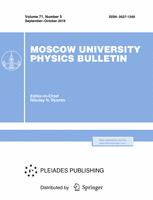Faculty of Physics
M.V.Lomonosov Moscow State University

# Measurement of NA62(CERN) sensitivity towards the search of $\pi^{0}\rightarrow3\gamma$ and $\pi^{0}\rightarrow4\gamma$ decays

## K. Yu. Gorshanov

### Memoirs of the Faculty of Physics 2023. N 4.

• Article
Annotation

The aim of the paper is measurement of NA62 experiment sensitivity towards the search for forbidden in Standard Model decay $\pi^{0}\rightarrow3\gamma$, that violates C-invariance principle, and allowed in Standard Model decay $\pi^{0}\rightarrow4\gamma$. Current upper limit on the probability of $\pi^{0}\rightarrow3\gamma$ ($\pi^{0}\rightarrow4\gamma$) decay is $3.1\times10^{-8}$ ($2\times10^{-8}$) with $90\%$ confidence level. In the paper $K^{+}\rightarrow\pi^{+}\pi^{0}$ decays are used as a source of $\pi^{0}$. The idea of selection of events with 3 products or 4 products after $\pi^{0}$ decay in NA62 detector was developed. NA62 detector and all physical processes were modelled with Monte Carlo method using Geant4. Single event sensitivity towards $\pi^{0}\rightarrow3\gamma$ ($\pi^{0}\rightarrow4\gamma$) decay without background events consideration is $\mathcal{O}(10^{-9})$, which is 1 order better than current upper limits. Consideration of background events for each decay leads to decline of sensitivity to the level of current limits.

Approved: 2023 August 5
PACS:
13.20.Cz Decays of π mesons
Authors
K. Yu. Gorshanov
$^1$Institute for Nuclear Research of the Russian Academy of Sciences (INR RAS)### Moscow University Physics Bulletin### Science News of the Faculty of Physics, Lomonosov Moscow State University

This new information publication, which is intended to convey to the staff, students and graduate students, faculty colleagues and partners of the main achievements of scientists and scientific information on the events in the life of university physicists.